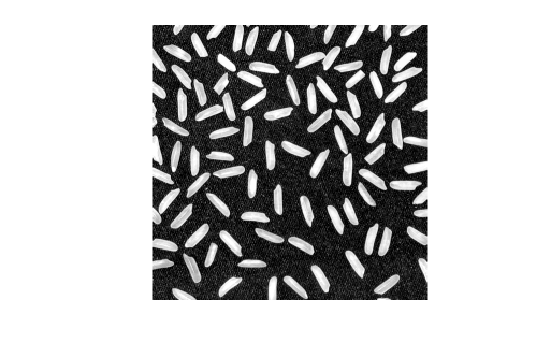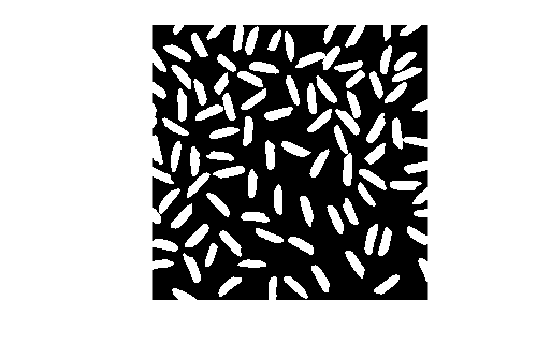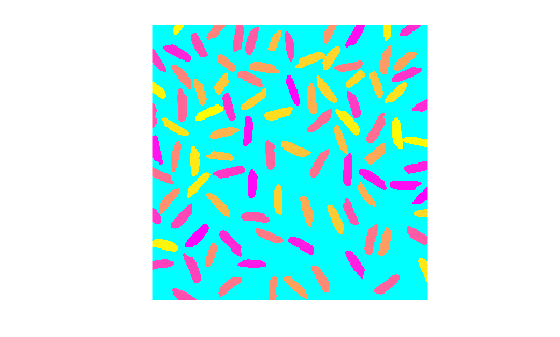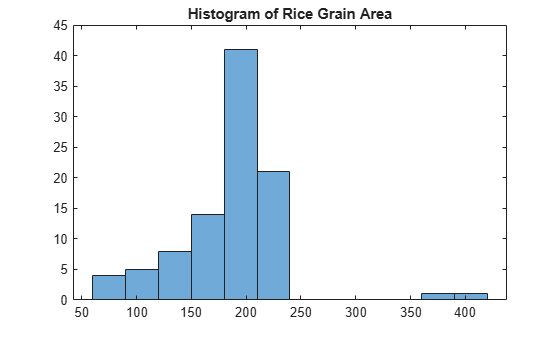# Correct Nonuniform Illumination and Analyze Foreground Objects

This example shows how to enhance an image as a preprocessing step before analysis. In this example, you correct the nonuniform background illumination and convert the image into a binary image to make it easy to identify foreground objects (individual grains of rice). You can then analyze the objects, such as finding the area of each grain of rice, and you can compute statistics for all objects in the image.

### Preprocess the Image

Read an image into the workspace.

```I = imread('rice.png'); imshow(I)```The background illumination is brighter in the center of the image than at the bottom. Preprocess the image to make the background illumination more uniform.

As a first step, remove all of the foreground (rice grains) using morphological opening. The opening operation removes small objects that cannot completely contain the structuring element. Define a disk-shaped structuring element with a radius of 15, which fits entirely inside a single grain of rice.

`se = strel('disk',15)`
```se = strel is a disk shaped structuring element with properties: Neighborhood: [29x29 logical] Dimensionality: 2 ```

To perform the morphological opening, use `imopen` with the structuring element.

```background = imopen(I,se); imshow(background)```Subtract the background approximation image, `background`, from the original image, `I`, and view the resulting image. After subtracting the adjusted background image from the original image, the resulting image has a uniform background but is now a bit dark for analysis.

```I2 = I - background; imshow(I2)```Use `imadjust` to increase the contrast of the processed image `I2` by saturating 1% of the data at both low and high intensities and by stretching the intensity values to fill the `uint8` dynamic range.

```I3 = imadjust(I2); imshow(I3)```Note that the prior two steps could be replaced by a single step using `imtophat` which first calculates the morphological opening and then subtracts it from the original image.

`I2 = imtophat(I,strel('disk',15));`

Create a binary version of the processed image so you can use toolbox functions for analysis. Use the `imbinarize` function to convert the grayscale image into a binary image. Remove background noise from the image with the `bwareaopen` function.

```bw = imbinarize(I3); bw = bwareaopen(bw,50); imshow(bw)```### Identify Objects in the Image

Now that you have created a binary version of the original image you can perform analysis of objects in the image.

Find all the connected components (objects) in the binary image. The accuracy of your results depends on the size of the objects, the connectivity parameter (4, 8, or arbitrary), and whether or not any objects are touching (in which case they could be labeled as one object). Some of the rice grains in the binary image `bw` are touching.

`cc = bwconncomp(bw,4)`
```cc = struct with fields: Connectivity: 4 ImageSize: [256 256] NumObjects: 95 PixelIdxList: {1x95 cell} ```
`cc.NumObjects`
```ans = 95 ```

View the rice grain that is labeled 50 in the image.

```grain = false(size(bw)); grain(cc.PixelIdxList{50}) = true; imshow(grain)```Visualize all the connected components in the image by creating a label matrix and then displaying it as a pseudocolor indexed image.

Use `labelmatrix` to create a label matrix from the output of `bwconncomp`. Note that `labelmatrix` stores the label matrix in the smallest numeric class necessary for the number of objects.

```labeled = labelmatrix(cc); whos labeled```
``` Name Size Bytes Class Attributes labeled 256x256 65536 uint8 ```

Use `label2rgb` to choose the colormap, the background color, and how objects in the label matrix map to colors in the colormap. In the pseudocolor image, the label identifying each object in the label matrix maps to a different color in an associated colormap matrix.

```RGB_label = label2rgb(labeled,'spring','c','shuffle'); imshow(RGB_label)```### Compute Area-Based Statistics

Compute the area of each object in the image using `regionprops`. Each rice grain is one connected component in the `cc` structure.

`graindata = regionprops(cc,'basic')`
```graindata=95×1 struct array with fields: Area Centroid BoundingBox ```

Create a new vector `grain_areas`, which holds the area measurement for each grain.

`grain_areas = [graindata.Area];`

Find the area of the 50th component.

`grain_areas(50)`
```ans = 194 ```

Find and display the grain with the smallest area.

`[min_area, idx] = min(grain_areas)`
```min_area = 61 ```
```idx = 16 ```
```grain = false(size(bw)); grain(cc.PixelIdxList{idx}) = true; imshow(grain)```Use the `histogram` command to create a histogram of rice grain areas.

```histogram(grain_areas) title('Histogram of Rice Grain Area')```